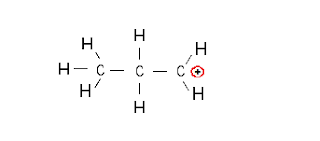Lewis configuration of the allylic carbocation | Chemistry Net

# Lewis configuration of the allylic carbocation

A simple procedure for writing Lewis Structures was given in a previous article entitled “Lewis Structures and the Octet Rule”. Several worked examples relevant to this procedure were given in previous posts please see the Sitemap - Table of Contents (Lewis Electron Dot Structures).

Let us consider the case of the allylic carbocation: C3H5+

Step 1: Connect the C atoms with single bonds and add H atoms so that an octet of electrons exists around each atom:Fig. 1:  The atoms connected with single bonds in the “initial” structure

Step 2:  Calculate the # of electrons in π bonds (multiple bonds) using  formula (1):

Where n in this case is 3, excluding the H atoms. Where V = (4 + 3 + 4 + 2 + 4 +2) – 1 = 18 , V is the number of valence electrons of the molecule.

Therefore, P = 6n + 2 – V = 6 * 3 + 2 – 18 = 2    So, there is one double bond in the molecule.

Step 3 & 4: The  resonance structures of the allylic carbocation are as follows:Fig. 2:  Resonance structures of the allylic carbocation

The two most plausible electron dot structures (ELDOTS) for the allylic carbocation are given in Fig. 2.